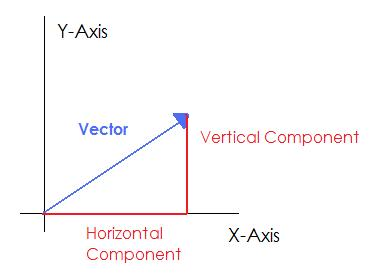Complimentary 1-hour tutoring consultation

MCAT Content / Translational Motion / Vectors Components

### Vectors, Components

Topic: Translational Motion

Vectors are geometric representations of magnitude and direction and can be expressed as arrows in two or three dimensions.

Vectors are geometric representations of magnitude and direction which are often represented by straight arrows, starting at one point on a coordinate axis and ending at a different point. All vectors have a length, called the magnitude, which represents some quality of interest so that the vector may be compared to another vector. Vectors, being arrows, also have a direction. This differentiates them from scalars, which are mere numbers without a direction.The original vector, defined relative to a set of axes. The horizontal component stretches from the start of the vector to its furthest x-coordinate. The vertical component stretches from the x-axis to the most vertical point on the vector. Together, the two components and the vector form a right triangle.

To visualize the process of decomposing a vector into its components, begin by drawing the vector from the origin of a set of coordinates. Next, draw a straight line from the origin along the x-axis until the line is even with the tip of the original vector. This is the horizontal component of the vector. To find the vertical component, draw a line straight up from the end of the horizontal vector until you reach the tip of the original vector. You should find you have a right triangle such that the original vector is the hypotenuse. Whenever you see motion at an angle, you should think of it as moving horizontally and vertically at the same time.

Practice Questions

Swine flu in Finland

MCAT Official Prep (AAMC)

Key Points

• Vectors can be broken down into two components: magnitude and direction.

• By taking the vector to be analyzed as the hypotenuse, the horizontal and vertical components can be found by completing a right triangle. The bottom edge of the triangle is the horizontal component and the side opposite the angle is the vertical component.

• The angle that the vector makes with the horizontal can be used to calculate the length of the two components.

Key Terms

Vector: A quantity having direction as well as magnitude, especially as determining the position of one point in space relative to another.

Scalars: A quantity having only magnitude, not direction. Physical quantities without a spatial direction

Displacement: A scalar quantity; an object’s change in position, only measuring from its starting position to the final position.

Coordinates: Numbers indicating a position with respect to some axis. For example, (x,y) coordinates indicate a position relative to the x and y axes.

Axis: An imaginary line around which an object spins or is symmetrically arranged.

Magnitude: A number assigned to a vector indicating its length.

Billing Information